## Rotational kinetic energy and angular momentum

6-9-98

### Rotational work and energy

Let's carry on madly working out equations applying to rotational motion by substituting the appropriate rotational variables into the straight-line motion equations. Work is force times displacement, so for rotation work must be torque times angular displacement: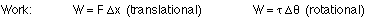A torque applied through a particular angular displacement does work. If the object is rotating clockwise and the torque is a clockwise torque, the work is positive; a counter-clockwise torque applied to a clockwise rotating object does negative work.

What about kinetic energy? A spinning object has rotational kinetic energy: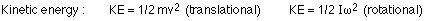A rolling object has both translational and rotational kinetic energy.

### Angular momentum

To finish off our comparison of translational (straight-line) and rotational motion, let's consider the rotational equivalent of momentum, which is angular momentum. For straight-line motion, momentum is given by p = mv. Momentum is a vector, pointing in the same direction as the velocity. Angular momentum has the symbol L, and is given by the equation:Angular momentum is also a vector, pointing in the direction of the angular velocity.

In the same way that linear momentum is always conserved when there is no net force acting, angular momentum is conserved when there is no net torque. If there is a net force, the momentum changes according to the impulse equation, and if there is a net torque the angular momentum changes according to a corresponding rotational impulse equation.

Angular momentum is proportional to the moment of inertia, which depends on not just the mass of a spinning object, but also on how that mass is distributed relative to the axis of rotation. This leads to some interesting effects, in terms of the conservation of angular momentum.

A good example is a spinning figure skater. Consider a figure skater who starts to spin with their arms extended. When the arms are pulled in close to the body, the skater spins faster because of conservation of angular momentum. Pulling the arms in close to the body lowers the moment of inertia of the skater, so the angular velocity must increase to keep the angular momentum constant.

### Parallels between straight-line motion and rotational motion

Let's take a minute to summarize what we've learned about the parallels between straight-line motion and rotational motion. Essentially, any straight-line motion equation has a rotational equivalent that can be found by making the appropriate substitutions (I for m, torque for force, etc.).### Example - Falling down

You've climbed up to the top of a 7.5 m high telephone pole. Just as you reach the top, the pole breaks at the base. Are you better off letting go of the pole and falling straight down, or sitting on top of the pole and falling down to the ground on a circular path? Or does it make no difference?

The answer depends on the speed you have when you hit the ground. The speed in the first case, letting go of the pole and falling straight down, is easy to calculate using conservation of energy: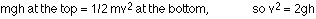In the second case, also apply conservation of energy. If you have negligible mass compared to the telephone pole, just work out the angular velocity of the telephone pole when it hits the ground. In this case we use rotational kinetic energy, and the height involved in the potential energy is half the length of the pole (which we can call h), because that's how much the center of gravity of the pole drops. So, for the second case: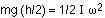For a uniform rod rotating about one end, the moment of inertia is 1/3 mL2. Solving for the angular velocity when the pole hits the ground gives: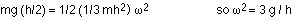For you, at the end of the pole, the velocity is h times the angular velocity, so: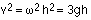So, if you hang on to the pole you end up falling faster than if you'd fallen under the influence of gravity alone. This also means that the acceleration of the end of the pole, just before the pole hits the ground, is larger than g (1.5 times as big, in this case), which is interesting.